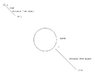# Fractal Nature of Gravitational Forces

• Lostpinky

#### Lostpinky

Hi All,

I'm no expert, nor have had any training, i just read a lot so I would be interested in some debate about an idea I have after reading lots of different things.

To try help explain the idea I've drawn a crude picture which is attached.

Can anyone explain to me the possibility of having a fractal nature to Gravity. I know gravity is a force and not matter therefore it cannot be directly fractal by itself but it may follow a law of power.

In the picture let's assume we take the force of gravity (G) as 1 on the surface of the Earth, or the moon, or the sun whatever macro body you like.

The we travel a certain distance away until G is 1/10th of surface gravity at which point G would be 1^-1.

Given the mass of the object affecting the actual value of G
EG: Earth G=9.98m/s^2, the moon G=1.625m/s^2
The distance from an object at which you reach 1^-1G would be proportional.

EG: For Earth at about 1.5 million km distance the sun's gravity overwhelms Earth's gravity

So let's say 10% Earth G is about 1350000km from Earth. I don't know if there is a name for this distance but let's call it the "0.1G line".

Now if the 0.1G line is proportional for all masses in the solar system let's move it down to the atomic level.

Imagine being on the surface of an electron or proton. you would feel 1G based on the mass of the particle. You're 0.1G line distance from the particle would be proportional to that of the Earth, depending on mass.

Blow it up further to the size of the galaxy. The 0.1G line would be a proportional distance from the Galactic mass.

Of course:
- Mass affects gravity.
- The more massive/dense the object the higher the gravitational field.

BUT

If you picture this sort of relationship you see that gravity on the largest scale is a power factor stronger than gravity at our scale, and that is powers stronger than gravity on the atomic level.

Is this type of law of powers application to gravity on several scales mathematically possible or am I misunderstanding things?

If it is possible then it follows a possible fractal pattern where gravity is the same at every level just orders of magnitude in difference.

If gravity drops away at a exponential rate then does it do so on both galactic and stellar scales?

#### Attachments

•fractalgrav.JPG
8 KB · Views: 417